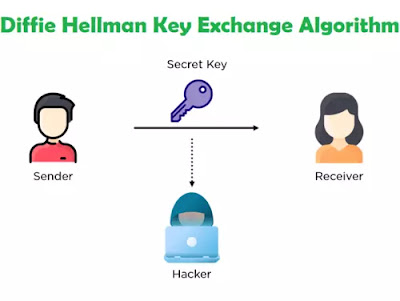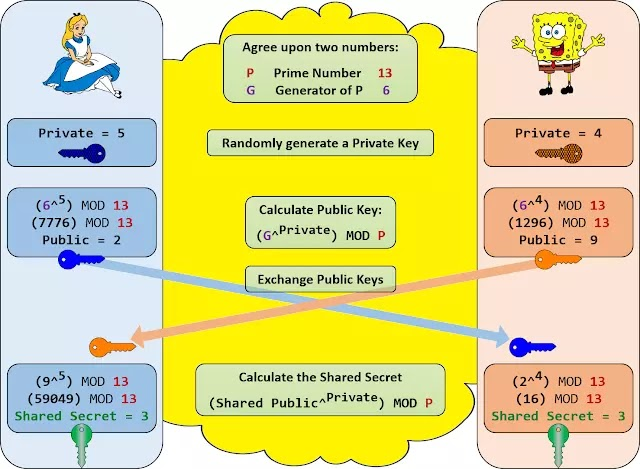# Diffie Hellman Key Exchange Algorithm Implementation in C

The Diffie-Hellman key exchange algorithm is a cryptographic method that securely exchanges cryptographic keys over an insecure communication channel. It was introduced by Whitfield Diffie and Martin Hellman in 1976 and is considered one of the fundamental concepts in modern cryptography. The algorithm allows two parties to agree upon a shared secret key without directly transmitting the key over the insecure channel.Diffie Hellman Key exchange algorithm

## Diffie Hellman Key exchange algorithm

The Diffie-Hellman key exchange algorithm is a method for securely exchanging keys over a public communication channel. It allows two parties to agree on a shared secret key without any prior secret information shared between them.Diffie Hellman Key exchange algorithm Implementation in C

It is primarily used to exchange cryptography keys for symmetric encryption algorithms. It was proposed in 1976 by Whitfield Diffie and Martin Hellman. Diffie-Hellman is currently used in many protocols like Secure Sockets Layer (SSL)/Transport Layer Security (TLS), Secure Shell (SSH), Internet Protocol Security (IPSec), and Public Key Infrastructure (PKI).

### Steps of Diffie Hellman key exchange Algorithm:

1       Requires two large numbers, one prime (P) and (G), a primitive root of P

2       P and G are both publicly available numbers

a.       P is at least 512 bits

3       Users pick private values a and b

4       Compute public values

a.       x = ga mod p

b.      y = GB mod p

5       Public values x and y are exchanged

6       Compute shared, private key

a.       ka = yamod p

b.      kb = xbmod p

c.       Algebraically, it can be shown that ka = kb

Users now have a symmetric secret key to encrypt.

### Example of Diffie Hellman Key exchange algorithm Implementation

1. Alice and Bob get public numbers
1. P = 23, G = 9
2. Alice and Bob compute public values
3. X = 94 mod 23 = 6561 mod 23 = 6
4. Y = 93 mod 23 = 729 mod 23    = 16
5. Alice and Bob exchange public numbers
2. Alice and Bob compute symmetric keys
1. ka = ya mod p = 164 mod 23 = 9
2. kb = xb mod p = 63 mod 23 = 9

3.       9 is the shared secret.

### The output of the Diffie Hellman Key exchange algorithm Implementation in C:

 Diffie Hellman Key exchange algorithm Implementation in C

### Summary:

The Diffie-Hellman key exchange algorithm is a secure method for exchanging keys over a public communication channel. It enables two parties to agree on a shared secret key without any prior confidential information shared between them. The algorithm is widely used in many protocols like SSL/TLS, SSH, IPSec, and PKI. Its implementation involves the use of large prime numbers and primitive roots and the computation of public and private values that are exchanged between the parties to generate a shared secret key. The algorithm's security is based on the difficulty of computing discrete logarithms.

#### RSA Algorithm(Encryption and Decryption) implementation in C

1.1.